Скачать презентацию Graph this assume motion along a line You

ea19ffe5f972097a5c766385024d04ab.ppt

• Количество слайдов: 11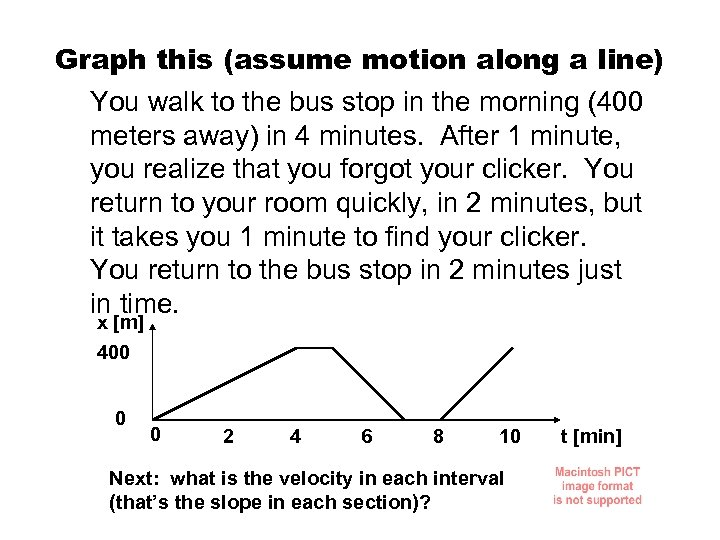Graph this (assume motion along a line) You walk to the bus stop in the morning (400 meters away) in 4 minutes. After 1 minute, you realize that you forgot your clicker. You return to your room quickly, in 2 minutes, but it takes you 1 minute to find your clicker. You return to the bus stop in 2 minutes just in time. x [m] 400 0 0 2 4 6 8 10 Next: what is the velocity in each interval (that’s the slope in each section)? t [min]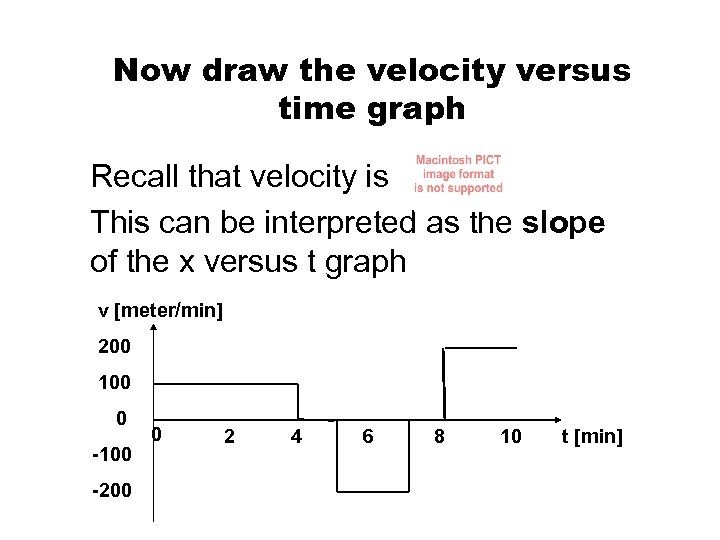Now draw the velocity versus time graph Recall that velocity is This can be interpreted as the slope of the x versus t graph v [meter/min] 200 100 0 -100 -200 0 2 4 6 8 10 t [min]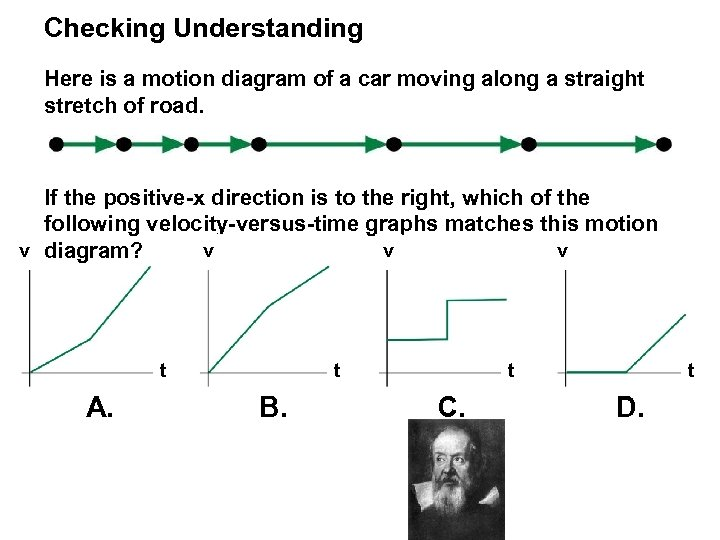Checking Understanding Here is a motion diagram of a car moving along a straight stretch of road. If the positive-x direction is to the right, which of the following velocity-versus-time graphs matches this motion v diagram? v v v t A. t B. t C. t D.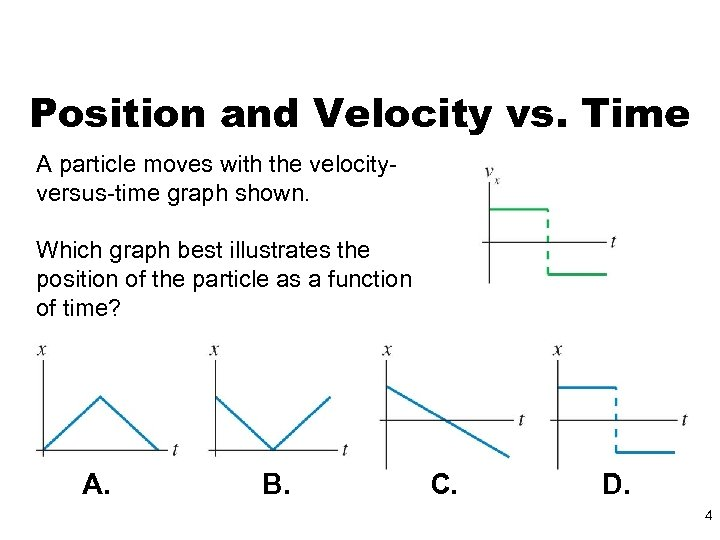Position and Velocity vs. Time A particle moves with the velocityversus-time graph shown. Which graph best illustrates the position of the particle as a function of time? A. B. C. D. 4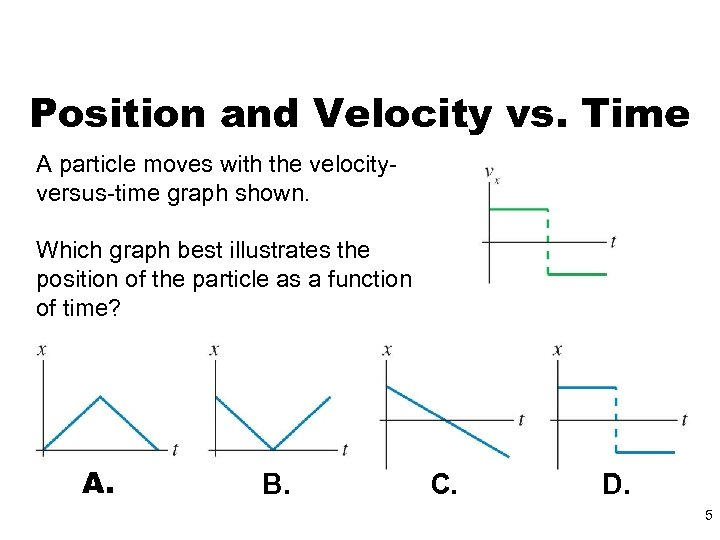Position and Velocity vs. Time A particle moves with the velocityversus-time graph shown. Which graph best illustrates the position of the particle as a function of time? A. B. C. D. 5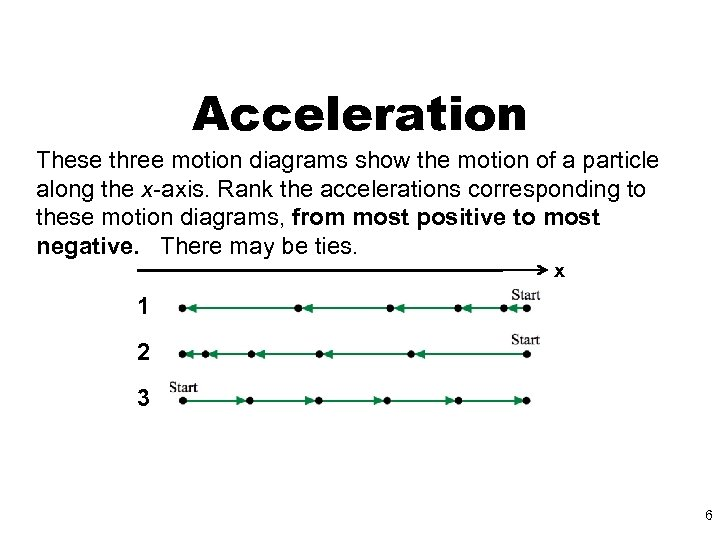Acceleration These three motion diagrams show the motion of a particle along the x-axis. Rank the accelerations corresponding to these motion diagrams, from most positive to most negative. There may be ties. x 1 2 3 6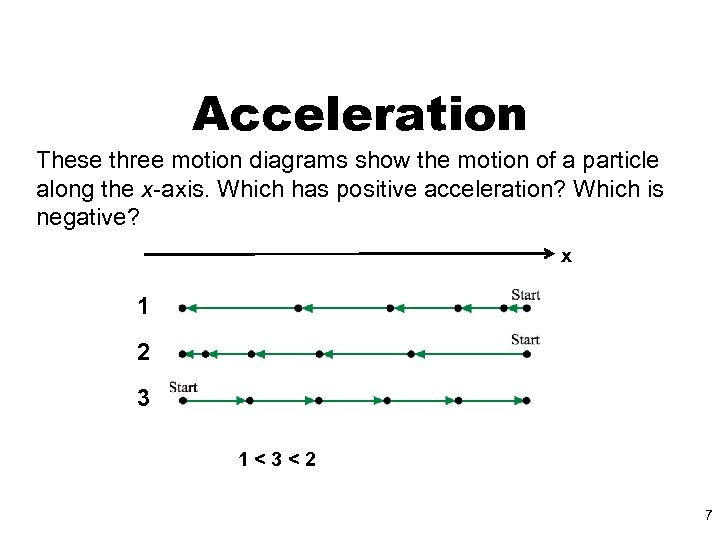Acceleration These three motion diagrams show the motion of a particle along the x-axis. Which has positive acceleration? Which is negative? x 1 2 3 1<3<2 7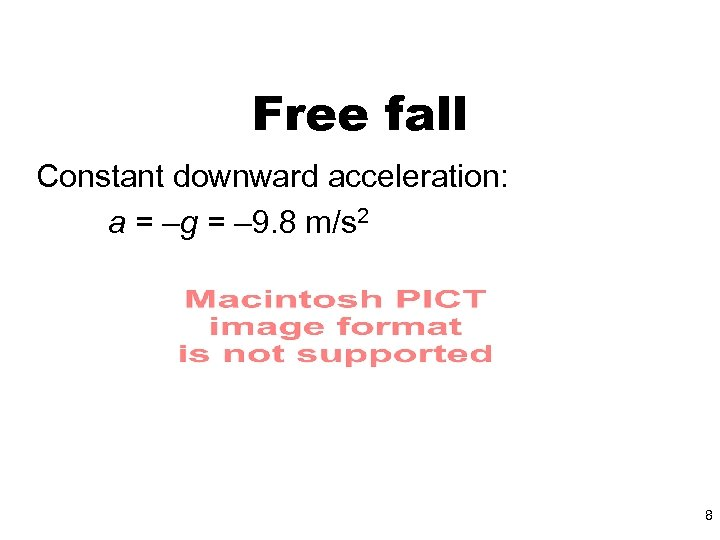Free fall Constant downward acceleration: a = –g = – 9. 8 m/s 2 8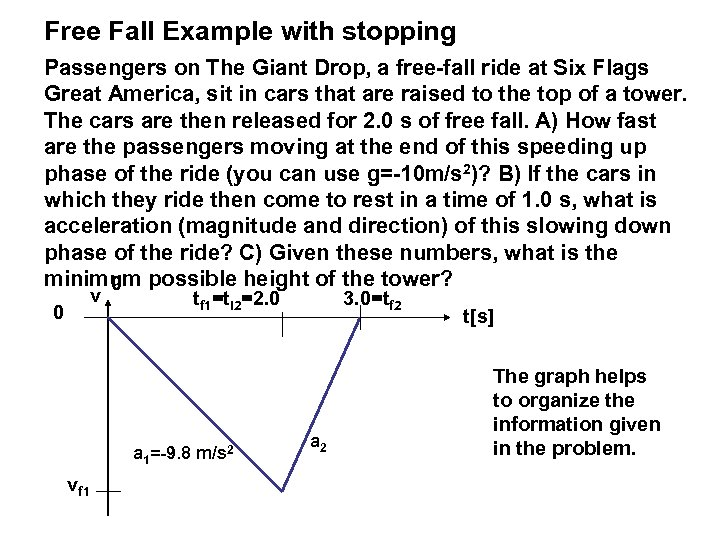Free Fall Example with stopping Passengers on The Giant Drop, a free-fall ride at Six Flags Great America, sit in cars that are raised to the top of a tower. The cars are then released for 2. 0 s of free fall. A) How fast are the passengers moving at the end of this speeding up phase of the ride (you can use g=-10 m/s 2)? B) If the cars in which they ride then come to rest in a time of 1. 0 s, what is acceleration (magnitude and direction) of this slowing down phase of the ride? C) Given these numbers, what is the minimum possible height of the tower? 0 0 v tf 1=ti 2=2. 0 a 1=-9. 8 m/s 2 vf 1 3. 0=tf 2 a 2 t[s] The graph helps to organize the information given in the problem.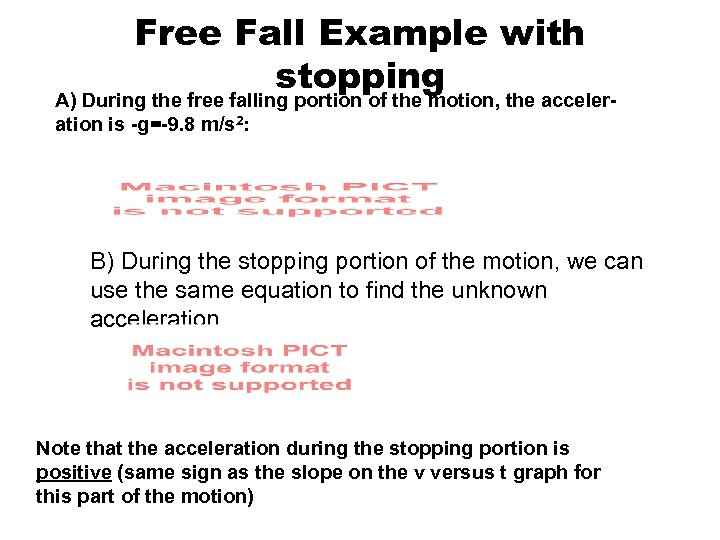Free Fall Example with stopping A) During the free falling portion of the motion, the acceleration is -g=-9. 8 m/s 2: B) During the stopping portion of the motion, we can use the same equation to find the unknown acceleration Note that the acceleration during the stopping portion is positive (same sign as the slope on the v versus t graph for this part of the motion)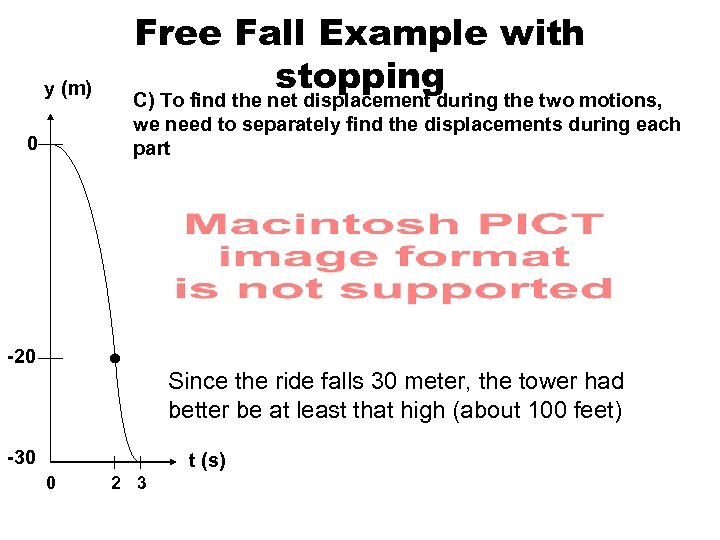y (m) 0 Free Fall Example with stopping C) To find the net displacement during the two motions, we need to separately find the displacements during each part -20 Since the ride falls 30 meter, the tower had better be at least that high (about 100 feet) -30 t (s) 0 2 3